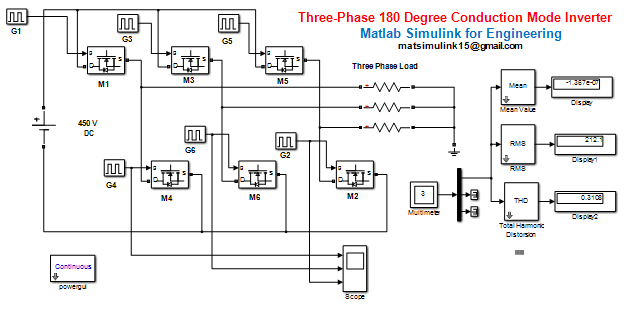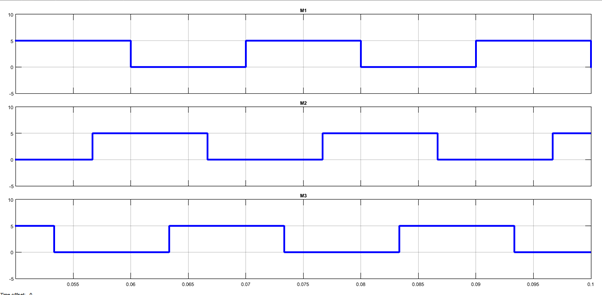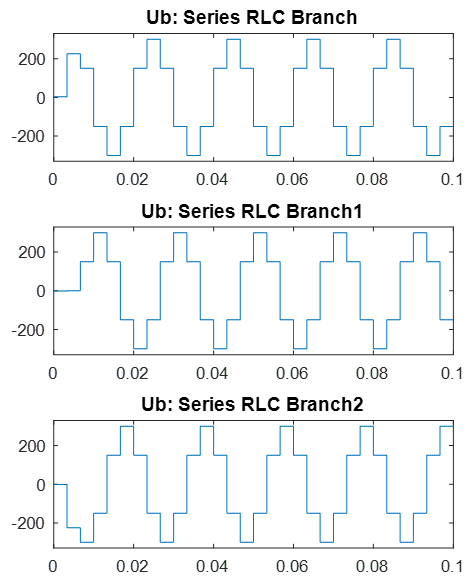3 Phase 180 Degree Mode Inverter using matlab simulink - Matlab Simulink for E & T

## Sunday, July 12, 2020180 Degree Conduction Mode Inverter using matlab simulink

# Three-Phase 180 Degree Conduction Mode Inverter# A three phase 180 degree conduction mode bridge type inverter model built up of six MOSFETs, 450 V DC input Supply , Star connected 300 ohm load, six pulse generator,. In this inverter, each MOSFET conducts for 180 degree and hence it is known as three phase VSI.

#### For best laptop for Matlab software-  https://amzn.to/39QeZ9dCamera- https://amzn.to/39KBi02Professional tripod- https://amzn.to/39Jg96w Angle (Degree) ON Switches OFF Switches 0°-60° M1, M5, M6 M2, M3, M4 60°-120° M1, M2, M6 M3, M4, M5 120°-180° M1, M2, M3 M4, M5, M6 180°-240° M2, M3, M4 M1, M5, M6 240°-300° M3, M4, M5 M1, M2, M6 300°-360° M4, M5, M6 M1, M2, M3 A period of 0.02 sec is taken so that the output voltage waveform is of frequency 50 Hz (T=1/f, i.e. 1/50=0.02). The pulse width of 50% is taken so that the MOSFET,  M1 conducts from 0 to 0.01 sec .i.e. 0 to 180 degree , as 0 to 0.02 sec is equivalent to 0 to 360 degree , the delay of 0.01 sec means 180 degree delay in electrical degree. Similarly the phase delay

for    G2 it is 0.01/3 sec,
for    G3 it is 0.02/3 sec,
for    G4 it is 0.01 sec.
for    G5 it is 0.04/3, and

# The gate pulse of switches M1, M3, M5 are shown in fig . it can observed from these gate pulse that each switch conducts for 0.01 sec, i.e. 180 degree.

####Gate Pulse of M1, M2 and M3The output voltage waveform of the three phases obtained after simulation the model is shown In  figure  .it can observer from figure that the first phase has phase angle of 0 degree , the second phase – 120 degree and third phase is 240 degree.

## FFT Analysis

for more details and if you want to complete model then contact to us.

Related Post –

# 3 Phase 120 Degree Mode Inverter using matlab simulink

For more matlab code and program

Please Like, Comment, follow our blog for new updates and Share and email subscribe for latest update

! THANK YOU !